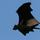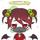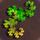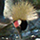## Social Question# Can you figure out the number of players in the tournament?

Asked by LostInParadise (27668) May 20th, 2012

It is getting warm and school is almost out, so I thought I would give a simple problem that is all insight and very little calculation.

An Internet tournament is being held for the board game Monopoly. If you do not like Monopoly, substitute any other game that can have four players. There will be four players per game. The tournament is single elimination. In each game, only the winner gets to play more games. There will be a total of 1365 games played. How many people are needed to register for the tournament? One simple multiplication and an even simpler addition will give the answer.

When I give the answer, I will show how the idea behind it can be used to give a completely intuitive derivation of the formula for geometric series, where each term has meaning. If you see where I am headed with this, feel free to jump in.

Observing members: 0Composing members: 0Yeah, it’s pretty simple. Each game eliminates 3 players, and each round of games eliminates ¾ of the total players. The simple addition is that at the end there is 1 player left. So… 1365 * 3 + 1 total players = 4096 (EDIT) players in the tournament.

But anyone who can play 6 rounds of Monopoly in a row is tougher than I am.

CWOTUS (26082)“Great Answer” (2) Flag as…Ok, here is my guess:
1364×3+4=4096

ragingloli (46984)“Great Answer” (2) Flag as…I second the motion for 1365*3+1=4096

PhiNotPi (12677)“Great Answer” (1) Flag as…PS, at first I tried thinking it through like cwotus, but I could not find a justification for anything I came up with.
So then I imagined a 3 round example with 1, 4, 16 games per round respectively. At the beginning there were 64 players (16×4) at 21 games. Then I devised an equation that would have 64 and 21 or a derivative thereof as constituents and 64 as a result.
The equation was 20×3 + 1×4=64.
I then tested that equation with a 4 round example (85 games, 256 players).

ragingloli (46984)“Great Answer” (0) Flag as…You guys are all good. Here is how to use this to derive the formula for geometric series, at least for natural numbers. I am going to use the value 4, but you can substitute any other natural number.

@ragingloli, You have provided the perfect segue.

There is only one way to hold a fair tournament, that is one where everyone would have to win the same number of games to win the tournament. It is similar to the way basketball and tennis tournaments are divided into rounds, except that there are four players instead of two. In the final round, there is just one game, In the semi-finals, there are four games, the winners of which go on to the final. Continuing this way, there are 4*4 = 16 games in the previous round, and so on. A tournament with n+1 rounds has 1 + 4 + 4^2 + ... + 4^n games. From the problem, we know that the total number of players is 3(1 + 4 + ... + 4^n) + 1. The number of players entering the tournament is also the number of players in the first round, which is 4^n games times 4 players per game, giving 4^(n+1) players. Therefore, 3(1 + 4 + ... + 4^n) + 1 = 4^(n+1), which leads immediately to the formula for geometric series, 1 + 4 + ... + 4^n = (4^(n+1) -1)/(4–1). Note that this is not an algebraic proof.

LostInParadise (27668)“Great Answer” (0) Flag as…3 losers per game x 1365 = ... my head hurts too much to do the number thingee.

Anyway. That’s the number.

6rant6 (13690)“Great Answer” (0) Flag as…@6rant6, After multiplying 3×1365, you have to add 1, for the one person who was not eliminated from any of the games, and who is consequently declared the winner.

LostInParadise (27668)“Great Answer” (0) Flag as…Well, um, it’s just that I’m here on Fluther, so obviously I am mostly interested in losers.

6rant6 (13690)“Great Answer” (0) Flag as…